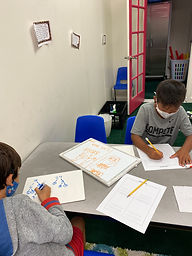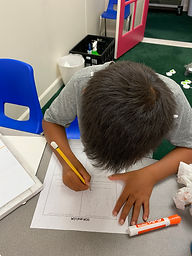## Ms. Kylie

### Target 1​

###### Lesson Type:

New

Number Operation

:

Integer Composition

Decompose numbers to determine the relationship between two whole numbers.

###### 1:

Decompose numbers into factors to gain numerical insight.

###### 2:

Identify the greatest common factor (GCF) of two whole numbers.

###### 3:

Compute the greatest common factor (GCF) using prime factorization.

###### 4:

Identify the lowest common multiple (LCM) of two whole numbers.

6th

###### Vocabulary:

Greatest Common Factor, Least Common Factor, Prime Number

Activities:

-Students were given two numbers and practiced finding the GCF using the factor tree diagram. Then they used the GCF and the remaining common prime factors to multiply and find the LCM.### Home Exploration

###### Guiding Questions:## Absent Students:

### Target 2

:

###### 1:

Define surface area as the measurement of the exterior layer of a figure.

###### 2:

Explain that surface area is the sum of the area of all the faces or surfaces of a figure.

###### 3:

Use cubes to determine the formula for calculating the surface area of rectangular prisms (2lw + 2lh + 2wh).

5th

###### Vocabulary:

-Surface Area, Area

Activities:

-Students were given the dimensions of a rectangular prism and used cubes to create the rectangular prism. Then they found the area of each side and added it all together to find the surface area.### Home Exploration

###### Guiding Questions:### Target 3

:

###### Vocabulary:

Activities:### Home Exploration